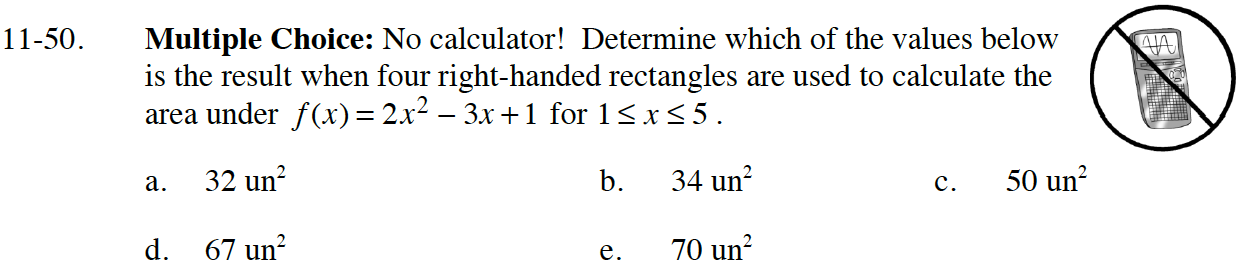### Home > CALC > Chapter 11 > Lesson 11.2.1 > Problem11-50

11-50.

Multiple Choice: No calculator! Determine which of the values below is the result when four right-handed rectangles are used to calculate the area under $f(x) = 2x^2 − 3x + 1$ for $1 ≤ x ≤ 5.$

1. $32$ un$^{2}$

1. $34$ un$^{2}$

1. $50$ un$^{2}$

1. $67$ un$^{2}$

1. $70$ un$^{2}$